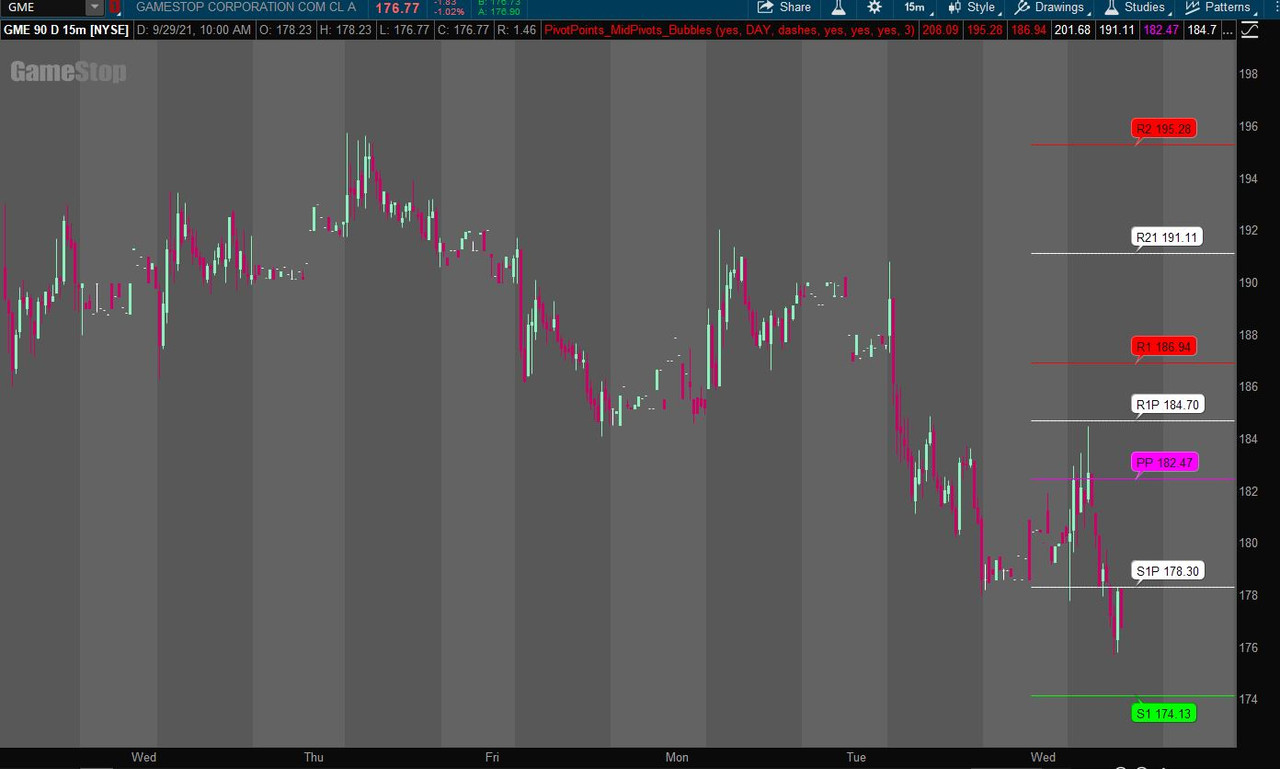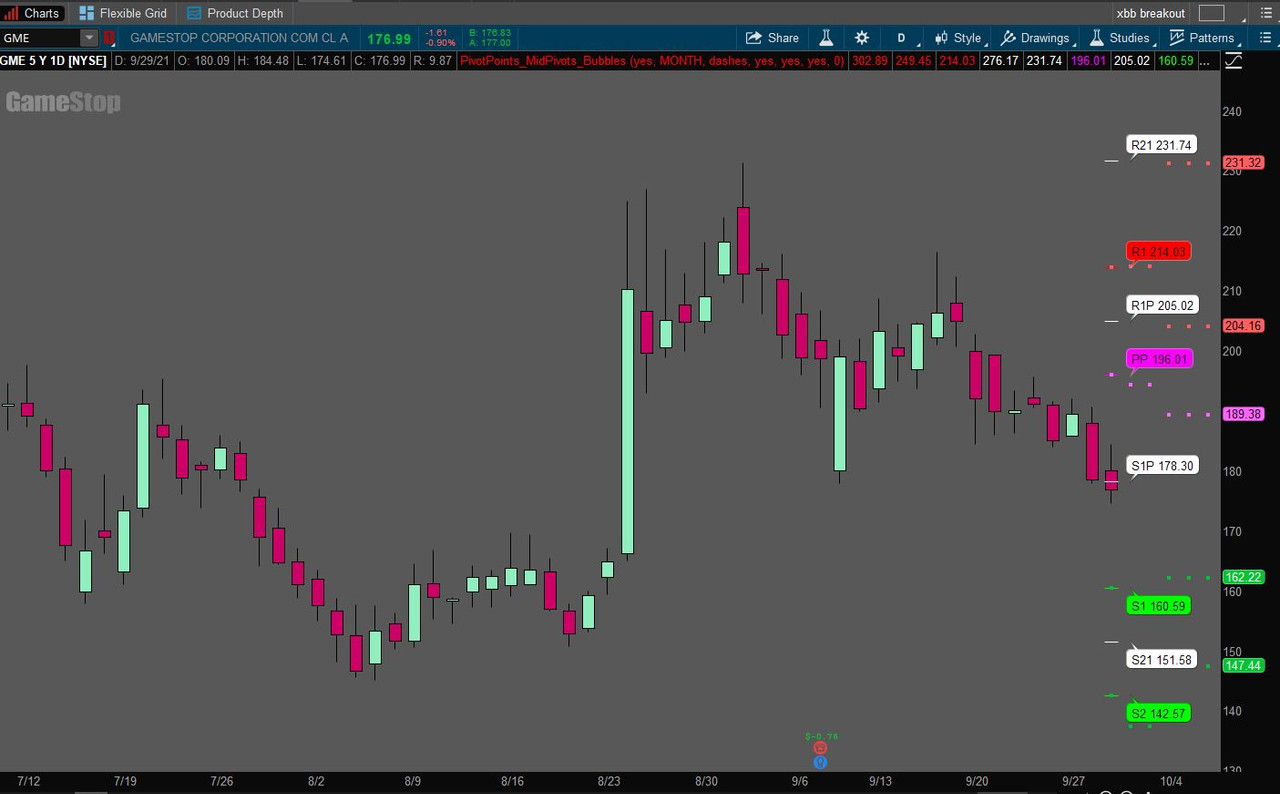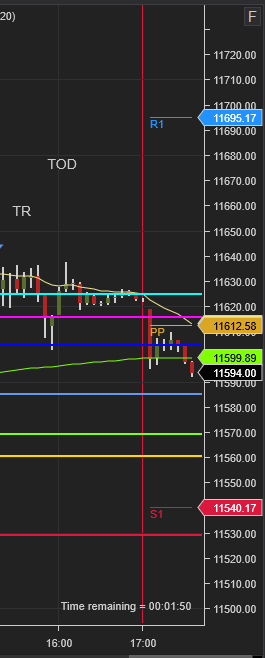# ToS Pivot Points Indicator for ThinkorSwim

#### anton

##### New member
Can anyone help add another pivot between each pivot? Like on a picture

Solution
Can anyone help add another pivot between each pivot? Like on a picture

This has a few options at the input screen on how to display the pivot points, including mid pivot points, bubbles, paintingstrategies, and etc.

The picture below is set to showtodayonly plots. The bubbles can be moved left/right. Make sure you have enough right expansion set at the chart setting timeaxis to display the bubbles.Ruby:
``````#
# TD Ameritrade IP Company, Inc. (c) 2011-2021
#
# Source code isn't available.

input showtodayonly           = yes;
input timeFrame               = {default "DAY", "WEEK", "MONTH"};
input lines                   = {default dashes, points, triangles, horizontal, squares};
input show_midpivots...``````
Can anyone help add another pivot between each pivot? Like on a picture

This has a few options at the input screen on how to display the pivot points, including mid pivot points, bubbles, paintingstrategies, and etc.

The picture below is set to showtodayonly plots. The bubbles can be moved left/right. Make sure you have enough right expansion set at the chart setting timeaxis to display the bubbles.Ruby:
``````#
# TD Ameritrade IP Company, Inc. (c) 2011-2021
#
# Source code isn't available.

input showtodayonly           = yes;
input timeFrame               = {default "DAY", "WEEK", "MONTH"};
input lines                   = {default dashes, points, triangles, horizontal, squares};
input show_midpivots          = yes;
input showbubbles_description = yes;
input showpricebubble         = yes;
def   today                   = GetDay() == GetLastDay();

plot R3   = if showtodayonly and !today then Double.NaN else PivotPoints().r3;
plot R2   = if showtodayonly and !today then Double.NaN else PivotPoints().r2;
plot R1   = if showtodayonly and !today then Double.NaN else PivotPoints().r1;
plot R32  = if !show_midpivots then Double.NaN else (R3 + R2) / 2;
plot R21  = if !show_midpivots then Double.NaN else (R2 + R1) / 2;
plot PP   = if showtodayonly and !today then Double.NaN else PivotPoints().pp;
plot R1P  = if !show_midpivots then Double.NaN else (R1 + PP) / 2;
plot S1   = if showtodayonly and !today then Double.NaN else PivotPoints().s1;
plot S1P  = if !show_midpivots then Double.NaN else (S1 + PP) / 2;
plot S2   = if showtodayonly and !today then Double.NaN else PivotPoints().s2;
plot S21  = if !show_midpivots then Double.NaN else (S2 + S1) / 2;
plot S3   = if showtodayonly and !today then Double.NaN else PivotPoints().s3;
plot S32  = if !show_midpivots then Double.NaN else (S3 + S2) / 2;

def paintingStrategy = if lines == lines.points then PaintingStrategy.POINTS else if lines == lines.triangles then PaintingStrategy.TRIANGLES else if lines == lines.dashes then PaintingStrategy.DASHES else if lines == lines.horizontal then PaintingStrategy.HORIZONTAL else PaintingStrategy.SQUARES;

R3.SetPaintingStrategy(paintingStrategy);
R32.SetPaintingStrategy(paintingStrategy);
R2.SetPaintingStrategy(paintingStrategy);
R21.SetPaintingStrategy(paintingStrategy);
R1.SetPaintingStrategy(paintingStrategy);
R1P.SetPaintingStrategy(paintingStrategy);
PP.SetPaintingStrategy(paintingStrategy);
S1P.SetPaintingStrategy(paintingStrategy);
S1.SetPaintingStrategy(paintingStrategy);
S21.SetPaintingStrategy(paintingStrategy);
S2.SetPaintingStrategy(paintingStrategy);
S32.SetPaintingStrategy(paintingStrategy);
S3.SetPaintingStrategy(paintingStrategy);

R3.SetDefaultColor(Color.RED);
R32.SetDefaultColor(Color.WHITE);
R2.SetDefaultColor(Color.RED);
R21.SetDefaultColor(Color.WHITE);
R1.SetDefaultColor(Color.RED);
R1P.SetDefaultColor(Color.WHITE);
PP.SetDefaultColor(Color.MAGENTA);
S1.SetDefaultColor(Color.GREEN);
S1P.SetDefaultColor(Color.WHITE);
S2.SetDefaultColor(Color.GREEN);
S21.SetDefaultColor(Color.WHITE);
S3.SetDefaultColor(Color.GREEN);
S32.SetDefaultColor(Color.WHITE);

#Bubbles to describe Pivot Levels

input bubblemover = 8;
def n = bubblemover;
def n1 = n + 1;

def StartPlot = if showbubbles_description == yes then (IsNaN(close[n]) and !IsNaN(close[n1])) else Double.NaN;

AddChartBubble(StartPlot, R3[n1], "R3 " + (if showpricebubble then AsText(R3[n1]) else ""), Color.RED,   if close[n1] > R3[n1] then no else yes);
AddChartBubble(StartPlot, R2[n1], "R2 " + (if showpricebubble then AsText(R2[n1]) else ""), Color.RED,   if close[n1] > R2[n1] then no else yes);
AddChartBubble(StartPlot, R1[n1], "R1 " + (if showpricebubble then AsText(R1[n1]) else ""), Color.RED,   if close[n1] > R1[n1] then no else yes);

AddChartBubble(StartPlot, PP[n1], "PP " + (if showpricebubble then AsText(PP[n1]) else ""), Color.MAGENTA,   if close[n1] > PP[n1] then no else yes);

AddChartBubble(StartPlot, S3[n1], "S3 " + (if showpricebubble then AsText(S3[n1]) else ""), Color.GREEN, if close[n1] > S3[n1] then no else yes);
AddChartBubble(StartPlot, S2[n1], "S2 " + (if showpricebubble then AsText(S2[n1]) else ""), Color.GREEN, if close[n1] > S2[n1] then no else yes);
AddChartBubble(StartPlot, S1[n1], "S1 " + (if showpricebubble then AsText(S1[n1]) else ""), Color.GREEN, if close[n1] > S1[n1] then no else yes);

AddChartBubble(StartPlot, R32[n1], "R32 " + (if showpricebubble then AsText(R32[n1]) else ""), Color.WHITE,   if close[n1] > R32[n1] then no else yes);
AddChartBubble(StartPlot, R21[n1], "R21 " + (if showpricebubble then AsText(R21[n1]) else ""), Color.WHITE,   if close[n1] > R2[n1] then no else yes);
AddChartBubble(StartPlot, R1P[n1], "R1P " + (if showpricebubble then AsText(R1P[n1]) else ""), Color.WHITE,   if close[n1] > R1[n1] then no else yes);
AddChartBubble(StartPlot, S32[n1], "S32 " + (if showpricebubble then AsText(S32[n1]) else ""), Color.WHITE, if close[n1] > S32[n1] then no else yes);
AddChartBubble(StartPlot, S21[n1], "S21 " + (if showpricebubble then AsText(S21[n1]) else ""), Color.WHITE, if close[n1] > S21[n1] then no else yes);
AddChartBubble(StartPlot, S1P[n1], "S1P " + (if showpricebubble then AsText(S1P[n1]) else ""), Color.WHITE, if close[n1] > S1P[n1] then no else yes);

R1.HideBubble();
R2.HideBubble();
R3.HideBubble();
PP.HideBubble();
S1.HideBubble();
S2.HideBubble();
S3.HideBubble();

R1P.HideBubble();
R21.HideBubble();
R32.HideBubble();
S1P.HideBubble();
S21.HideBubble();
S32.HideBubble();``````

This has a few options at the input screen on how to display the pivot points, including mid pivot points, bubbles, paintingstrategies, and etc.

The picture below is set to showtodayonly plots. The bubbles can be moved left/right. Make sure you have enough right expansion set at the chart setting timeaxis to display the bubbles.
thanks, but your pivot does not match the one I need

thanks, but your pivot does not match the one I need

I think they match now. What you are requesting is an addition to TOS's proprietary pivotpoints indicator. What I used was a workaround by referencing the individual points from that script.

I have now made the timeframe reference incorporated in those points, whereas before, it was just "DAY"#
# TD Ameritrade IP Company, Inc. (c) 2011-2021
#
# Source code isn't available.

input showtodayonly = yes;
input timeFrame = {default "DAY", "WEEK", "MONTH"};
input lines = {default dashes, points, triangles, horizontal, squares};
input show_midpivots = yes;
input showbubbles_description = yes;
input showpricebubble = yes;
def today = GetDay() == GetLastDay();

plot R3 = if showtodayonly and !today then Double.NaN else PivotPoints("time frame" = timeframe);
plot R2 = if showtodayonly and !today then Double.NaN else PivotPoints("time frame" = timeframe).r2;
plot R1 = if showtodayonly and !today then Double.NaN else PivotPoints("time frame" = timeframe).R1;
plot R32 = if !show_midpivots then Double.NaN else (R3 + R2) / 2;
plot R21 = if !show_midpivots then Double.NaN else (R2 + R1) / 2;
plot PP = if showtodayonly and !today then Double.NaN else PivotPoints("time frame" = timeframe).PP;
plot R1P = if !show_midpivots then Double.NaN else (R1 + PP) / 2;
plot S1 = if showtodayonly and !today then Double.NaN else PivotPoints("time frame" = timeframe).S1;
plot S1P = if !show_midpivots then Double.NaN else (S1 + PP) / 2;
plot S2 = if showtodayonly and !today then Double.NaN else PivotPoints("time frame" = timeframe).S2;
plot S21 = if !show_midpivots then Double.NaN else (S2 + S1) / 2;
plot S3 = if showtodayonly and !today then Double.NaN else PivotPoints("show only today" = showtodayonly, "time frame" = timeframe).S3;
plot S32 = if !show_midpivots then Double.NaN else (S3 + S2) / 2;

def paintingStrategy = if lines == lines.points then PaintingStrategy.POINTS else if lines == lines.triangles then PaintingStrategy.TRIANGLES else if lines == lines.dashes then PaintingStrategy.DASHES else if lines == lines.horizontal then PaintingStrategy.HORIZONTAL else PaintingStrategy.SQUARES;

R3.SetPaintingStrategy(paintingStrategy);
R32.SetPaintingStrategy(paintingStrategy);
R2.SetPaintingStrategy(paintingStrategy);
R21.SetPaintingStrategy(paintingStrategy);
R1.SetPaintingStrategy(paintingStrategy);
R1P.SetPaintingStrategy(paintingStrategy);
PP.SetPaintingStrategy(paintingStrategy);
S1P.SetPaintingStrategy(paintingStrategy);
S1.SetPaintingStrategy(paintingStrategy);
S21.SetPaintingStrategy(paintingStrategy);
S2.SetPaintingStrategy(paintingStrategy);
S32.SetPaintingStrategy(paintingStrategy);
S3.SetPaintingStrategy(paintingStrategy);

R3.SetDefaultColor(Color.RED);
R32.SetDefaultColor(Color.WHITE);
R2.SetDefaultColor(Color.RED);
R21.SetDefaultColor(Color.WHITE);
R1.SetDefaultColor(Color.RED);
R1P.SetDefaultColor(Color.WHITE);
PP.SetDefaultColor(Color.MAGENTA);
S1.SetDefaultColor(Color.GREEN);
S1P.SetDefaultColor(Color.WHITE);
S2.SetDefaultColor(Color.GREEN);
S21.SetDefaultColor(Color.WHITE);
S3.SetDefaultColor(Color.GREEN);
S32.SetDefaultColor(Color.WHITE);

#Bubbles to describe Pivot Levels

input bubblemover = 1;
def n = bubblemover;
def n1 = n + 1;

def StartPlot = if showbubbles_description == yes then (IsNaN(close[n]) and !IsNaN(close[n1])) else Double.NaN;

AddChartBubble(StartPlot, R3[n1], "R3 " + (if showpricebubble then AsText(R3[n1]) else ""), Color.RED, if close[n1] > R3[n1] then no else yes);
AddChartBubble(StartPlot, R2[n1], "R2 " + (if showpricebubble then AsText(R2[n1]) else ""), Color.RED, if close[n1] > R2[n1] then no else yes);
AddChartBubble(StartPlot, R1[n1], "R1 " + (if showpricebubble then AsText(R1[n1]) else ""), Color.RED, if close[n1] > R1[n1] then no else yes);

AddChartBubble(StartPlot, PP[n1], "PP " + (if showpricebubble then AsText(PP[n1]) else ""), Color.MAGENTA, if close[n1] > PP[n1] then no else yes);

AddChartBubble(StartPlot, S3[n1], "S3 " + (if showpricebubble then AsText(S3[n1]) else ""), Color.GREEN, if close[n1] > S3[n1] then no else yes);
AddChartBubble(StartPlot, S2[n1], "S2 " + (if showpricebubble then AsText(S2[n1]) else ""), Color.GREEN, if close[n1] > S2[n1] then no else yes);
AddChartBubble(StartPlot, S1[n1], "S1 " + (if showpricebubble then AsText(S1[n1]) else ""), Color.GREEN, if close[n1] > S1[n1] then no else yes);

AddChartBubble(StartPlot, R32[n1], "R32 " + (if showpricebubble then AsText(R32[n1]) else ""), Color.WHITE, if close[n1] > R32[n1] then no else yes);
AddChartBubble(StartPlot, R21[n1], "R21 " + (if showpricebubble then AsText(R21[n1]) else ""), Color.WHITE, if close[n1] > R2[n1] then no else yes);
AddChartBubble(StartPlot, R1P[n1], "R1P " + (if showpricebubble then AsText(R1P[n1]) else ""), Color.WHITE, if close[n1] > R1[n1] then no else yes);
AddChartBubble(StartPlot, S32[n1], "S32 " + (if showpricebubble then AsText(S32[n1]) else ""), Color.WHITE, if close[n1] > S32[n1] then no else yes);
AddChartBubble(StartPlot, S21[n1], "S21 " + (if showpricebubble then AsText(S21[n1]) else ""), Color.WHITE, if close[n1] > S21[n1] then no else yes);
AddChartBubble(StartPlot, S1P[n1], "S1P " + (if showpricebubble then AsText(S1P[n1]) else ""), Color.WHITE, if close[n1] > S1P[n1] then no else yes);

R1.HideBubble();
R2.HideBubble();
R3.HideBubble();
PP.HideBubble();
S1.HideBubble();
S2.HideBubble();
S3.HideBubble();

R1P.HideBubble();
R21.HideBubble();
R32.HideBubble();
S1P.HideBubble();
S21.HideBubble();
S32.HideBubble();

•anton
Hey @SleepyZ
Can you also modify the code for Pivot Points to painting strategy , show bubbles and within the bubbles whether to show the price like you did for the other Pivot? I tried modiying the code as per your logic, but it didnt return anything.

#
# TD Ameritrade IP Company, Inc. (c) 2011-2021
#
# Source code isn't available.

input showOnlyToday = No;
input timeFrame = {default "DAY", "WEEK", "MONTH"};

plot R3 = Double.NaN;
plot R2 = Double.NaN;
plot R1 = Double.NaN;
plot PP = Double.NaN;
plot S1 = Double.NaN;
plot S2 = Double.NaN;
plot S3 = Double.NaN;

Thanks for the quick response bro. Have you tested this study? What has your experience been?
I did test the study. It is not traditional pivots, but TOS's proprietary pivot study, which I mearly referenced. I do not use pivot studies in my trading, but others find them useful.

•thealphabreed
I did test the study. It is not traditional pivots, but TOS's proprietary pivot study, which I mearly referenced. I do not use pivot studies in my trading, but others find them useful.
I am testing TOS Pivots vs Camarilla to see which works better/

Hi Team,

This is the original TOS PivotPoint Indicator (pink doted line shown in image) and was wondering if there is anyway possible this indicator does not plot on the extended side of the chart as a continuation, or if we can choose to have this indicator plot only as an expansion or not. Thanks in advance.

input showOnlyToday = No;
input timeFrame = {default "DAY", "WEEK", "MONTH"};

plot R3 = Double.NaN;
plot R2 = Double.NaN;
plot R1 = Double.NaN;
plot PP = Double.NaN;
plot S1 = Double.NaN;
plot S2 = Double.NaN;
plot S3 = Double.NaN;

Hello,

Need Pivot Point/Support Resistance Study that will show as indicated in the image below. Study based on same data as the TOS stock Pivot study from the library however I only want lines in immediate right side of chart along with text indicator, i.e. R1, R2, P, S1, etc. and only extend for a short length. Restriction with stock study is it shows lines for duration of the day which do not want. Hopefully I can edit study if I want lines longer, shorter, different color, etc.

Secondly, while here is there any way to "download' so to speak thinkscript code from existing studies to use as basis for new study rather than build from scratch, kind of similar to the scenario above. Thank you.Hi Team,

This is the original TOS PivotPoint Indicator (pink doted line shown in image) and was wondering if there is anyway possible this indicator does not plot on the extended side of the chart as a continuation, or if we can choose to have this indicator plot only as an expansion or not. Thanks in advance.

input showOnlyToday = No;
input timeFrame = {default "DAY", "WEEK", "MONTH"};

plot R3 = Double.NaN;
plot R2 = Double.NaN;
plot R1 = Double.NaN;
plot PP = Double.NaN;
plot S1 = Double.NaN;
plot S2 = Double.NaN;
plot S3 = Double.NaN;

Hello,

Need Pivot Point/Support Resistance Study that will show as indicated in the image below. Study based on same data as the TOS stock Pivot study from the library however I only want lines in immediate right side of chart along with text indicator, i.e. R1, R2, P, S1, etc. and only extend for a short length. Restriction with stock study is it shows lines for duration of the day which do not want. Hopefully I can edit study if I want lines longer, shorter, different color, etc.

Secondly, while here is there any way to "download' so to speak thinkscript code from existing studies to use as basis for new study rather than build from scratch, kind of similar to the scenario above. Thank you.### Not the exact question you're looking for?

87k+ Posts
238 Online## The Market Trading Game Changer

Join 2,500+ subscribers inside the useThinkScript VIP Membership Club
• Exclusive indicators
• Proven strategies & setups
• Private Discord community
• Exclusive members-only content
• 1 full year of unlimited support

What is useThinkScript?

useThinkScript is the #1 community of stock market investors using indicators and other tools to power their trading strategies. Traders of all skill levels use our forums to learn about scripting and indicators, help each other, and discover new ways to gain an edge in the markets.

How do I get started?

We get it. Our forum can be intimidating, if not overwhelming. With thousands of topics, tens of thousands of posts, our community has created an incredibly deep knowledge base for stock traders. No one can ever exhaust every resource provided on our site.

If you are new, or just looking for guidance, here are some helpful links to get you started.

What are the benefits of VIP Membership?